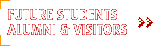2001 Technical Reports

## An Algorithm for Quantitative Verification of Probabilistic Transition Systems

### Franck van Breugel and James Worrell

Technical Report CS-2001-01

York University

April 2001

Abstract

This paper is concerned with a notion of behavioral equivalence for reactive probabilistic transition systems as arise, say, in the operational semantics of probabilistic process calculi. We solve a domain equation in the category of metric spaces and nonexpansive maps involving a probabilistic powerdomain functor based on the Hutchinson metric on probability measures. The resultant domain can be considered as the space of bisimulation equivalence classes of (possibly continuous state) probabilistic processes. Furthermore, the metric on this space induces a natural pseudometric on the class of all probabilistic transition systems. This can be considered as a quantitative notion of behavioural equivalence for probabilistic processes which varies smoothly with respect to transition probabilities. Our main contribution is to give an algorithm to calculate the distance between finite state processes in polynomial time. The body of the main loop of the algorithm involves a linear optimization: the so-called transshipment problem.